# GSEB Solutions Class 6 Maths Chapter 5 Understanding Elementary Shapes Ex 5.1

Gujarat Board GSEB Textbook Solutions Class 6 Maths Chapter 5 Understanding Elementary Shapes Ex 5.1 Textbook Questions and Answers.

## Gujarat Board Textbook Solutions Class 6 Maths Chapter 5 Understanding Elementary Shapes Ex 5.1

Question 1.
What is the disadvantage in comparing line segments by mere observation?
Solution:
Comparing the line segments simply by ‘observation’ may not be accurate. For example, the line segments AB and CD (in the following figure) seem to be equal, but actually they are not.Question 2.
Why is it better use a divider than a ruler while measuring the length of a line segment?
Solution:
Measuring the length of a line segment using a ruler may accompany with some errors due to:
(i) Thickness of the ruler
(ii) Angular viewing
These errors can be removed by measuring a line segment with the help of a divider.
So, the use of a divider is better than a ruler.

Question 3.
Draw any line segment, say AB. Take any point C lying in between A and B. Measure the: lengths of AB, BC and AC. Is AB = AC + CB ?
Note: If A, B, C are any three points on a line such that AC + CB = AB, then we can be sure that C lies between A and B.
Solution:
Measuring the lengths of the segments AB, BC and AC, we have: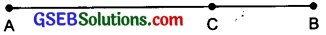$$\overline{\mathrm{AC}}$$ = 6.3 cm
$$\overline{\mathrm{BC}}$$ = 2.7 cm
$$\overline{\mathrm{AB}}$$ = 9.0 cm
AC + BC = 6.3 cm + 2.7 cm = 9.0 cm and AB = 9.0 cm
AC + BC = AB Hence verified.Question 4.
If A, B, C are three points on a line such that AB = 5 cm, BC = 3 cm and AC 8 cm, which one of them lies between the other two?
Solution: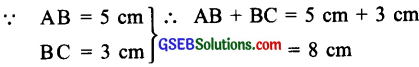But AC = 8cm
The point B lies between A and C.

Question 5.
Verify, whether D is the mid-point of $$\overline{\mathrm{AG}}$$.
Solution:
Since,
AG = 7 cm – 1 cm = 6 cm
AD = 4cm – 1cm = 3cm
DG = 7cm – 4cm = 3cmSo, D is the mid-point of AG.Question 6.
If B is the mid-point of and C is the mid-point of I5. where A, B, C, D lie on a straight line, say why AB = CD?
Solution:
Since, B is the mid-point of AC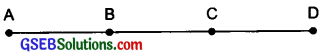AB = BC ………….(i)
Similarly, C is the mid-point of BD
BC = CD ………………(ii)
From (i) and (ii),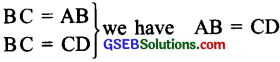Question 7.
Draw five triangles and measure their sides. Check in each case, if the sum of the lengths of any two sides is always less than the third side.
Solution:
We draw triangles with different set of lengths:
(i) In ∆ABC, we have
AB = 3.7 cm,
BC = 3.7 cm
and AC = 3.7 cm
= AB + BC
= 3.7 cm + 3.7 cm
= 74cm
7.4 > 3.7
(AB + BC) > AC
Similarly, we can have,
(BC + AC) > AB
(AB + AC) > BC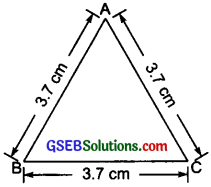(ii) In ∆PQR, we have:
PQ = 4cm,
PR = 2.7 cm
and RQ = 4.7cm
= PQ + RQ
= 4cm + 4.7cm
a = 8.7 cm
PQ + PR = 4 cm + 2.7 cm = 6.7 cm
RQ + PR = 2.7 cm + 4.7 cm = 7.4 cm
(PQ + RQ) > PR
(PQ + PR) > RQ
(RQ + PR) > PQ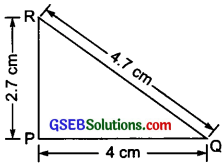(iii) In ∆BCD, we have
BC = 3 cm,
CD = 4 cm and
BD = 5 cm
Now,
= BC + CD
= 3 cm + 4 cm = 7
= BD + CD = 5 cm +4 cm = 9 cmBC + BD = 3 cm + 5 cm = 8 cm
(BC + CD) > BD
(BD + CD) > BC
(BC + BD) > CD

(iv) In ∆XYZ, we haveXY = 4.5 cm,
YZ = 3.8cm
and XZ = 2cm
XY + YZ = 4.5 cm + 3.8 cm = 8.3 cm
YZ + XZ = 3.8 cm +2 cm = 5.8 cm
XZ + XY = 2 cm + 4.5 cm = 6.5 cm
(XY + YZ) > XZ
(YZ + XZ) > XY
(XZ + XY) > YZ

(v) In ∆KLM, we have: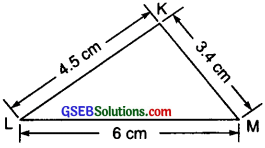KL = 4.5 cm, KM = 3.4 cm and LM = 6 cm
KL + KM = 4.5cm + 3.4cm = 7.9 cm
KM + LM = 3.4 cm + 6 cm = 9.4 cm
KL + LM = 4.5 cm + 6 cm = 10.5 cm
(KL + KM) > LM
(KM + LM) > KL
and (KL + LM) > KM.
In each of the above cases, we have seen that the sum of the lengths of any two sides is always greater than the third side.
Thus, the sum of the lengths of any two sides of a triangle is never less than its third side.